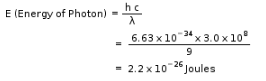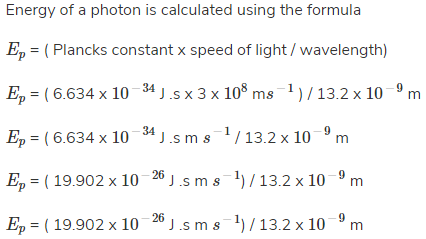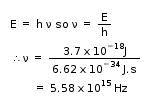Courses

# Test: Planck’s Quantum Theory

## 15 Questions MCQ Test Chemistry for JEE | Test: Planck’s Quantum Theory

Description
This mock test of Test: Planck’s Quantum Theory for JEE helps you for every JEE entrance exam. This contains 15 Multiple Choice Questions for JEE Test: Planck’s Quantum Theory (mcq) to study with solutions a complete question bank. The solved questions answers in this Test: Planck’s Quantum Theory quiz give you a good mix of easy questions and tough questions. JEE students definitely take this Test: Planck’s Quantum Theory exercise for a better result in the exam. You can find other Test: Planck’s Quantum Theory extra questions, long questions & short questions for JEE on EduRev as well by searching above.
QUESTION: 1

### The energy of a photon in Joules that has a wavelength of 9.0 m is:

Solution:QUESTION: 2

### The ratio of the energy of a photon of 2000 Çº wavelength radiation to that of 4000 Çº radiation is:

Solution:

We have E = hc/λ
E1/E2 = 4000Å/2000Å = 2

QUESTION: 3

### A radio operator broadcast on the 6-meter band. The frequency of this electromagnetic radiation is __________ MHz.

Solution:

The speed of all form of electromagnetic wave is related by the equation c = λ.ν,

Where c is the speed of light = 3.00 x 108 m/s, λ is the wavelength in m and ν is the frequency is s-1 or Hz.

Therefore, ν = 3.0 x108 / 6 = 5 x 108 s-1 = 50 MHz

QUESTION: 4

Electromagnetic radiation travels through vacuum at a speed of __________ m/s.

Solution:

The speed of light (electromagnetic radiation) through vacuum has a constant value of 3.00 x 108m/s and is independent of the wavelength in vacuum.

QUESTION: 5

What is the frequency of light in s-1 that has a wavelength of 3.12 x 10-3 cm?

Solution:

The wavelength (λ) and frequency (ν) of electromagnetic wave is related by the equation c = λ.ν,

Where c is the speed of light = 3.00 x 108 m/s, λ is the wavelength in m and ν is the frequency is s-1or Hz.

Substituting the values in the formula, we get,

c = λ.ν

ν = c/λ

ν = 3.0 x 108 / 3.12 x 10-5

= 0.96 x 1013 m =  9.62 X 1012

QUESTION: 6

The ejected electrons from the surface of metal in photoelectric effect are called:

Solution:

If light beyond a specific threshold frequency hits a metal surface, electrons are ejected from the surface of the metal. The minimum energy required to eject an electron from the surface is called the work function of the metal. These ejected electrons are called photoelectrons. The kinetic energy of the most energetic electron among these photoelectrons is called the maximum kinetic energy.

QUESTION: 7

If the value of azimuthal quantum number is 3, the possible values of magnetic quantum numbers would be:

Solution:

The total possible values of m for l range from – through 0 to +.

QUESTION: 8

What is the energy of a photon that has a wavelength of 13.2 nm?

Solution:= 1.507 x 10-15J.

QUESTION: 9

What is correct about wave number?

Solution:

Wavenumber is defined as the number of complete wave cycles per unit length of a linear space. It can also be referred to as the spatial frequency of the waves represented in radians per unit distance, or cycles per unit distance. It is usually measured in units of reciprocal centimeters (cm-1) and reciprocal meters (m-1).

QUESTION: 10

The wavelength of light that has a frequency of 1.20 × 1013s-1 is __________ m.

Solution:

The speed of all form of electromagnetic wave is related by the equation c = λ.v, whereby, λ = 3.0 x 108 / 1.2 x 1013 = 2.5 x 10 -5 m.

QUESTION: 11

Which of the following radiation has the shortest wavelength.

Solution:

Radio waves, infrared rays, visible light, ultraviolet rays, X-rays, and gamma raysare all types of electromagnetic radiation. Radio waves have the longest wavelength, and gamma rays have the shortest wavelength.

QUESTION: 12

Who discovered and first used the constant h = 6.6 x 10-34 J.s?

Solution:

Max Planck gave the Planck’s quantum theory and was the first to determine the value of h which is the Planck’s constant.

QUESTION: 13

The energy of a photon of light has what kind of proportionality to its frequency and its wavelength.

Solution:

As E=h(frequency) E is directly proportional to frequency and as frequency = speed of light / wavelength frequency is inversely proportional to wavelength.

QUESTION: 14

What is the wavelength of light (nm) that has a frequency 4.62 ×1014s-1?

Solution:

The wavelength (λ) and frequency (ν) of electromagnetic wave is related by the equation c = λ.ν, whereby, λ = 3.0 x108 / 4.62 ×1014 =  0.649 x 10-6 m = 649nm

QUESTION: 15

What is the frequency of a photon that has an energy of 3.7 x 10-18J?

Solution: